JAVA语言之10道简单算法题白羽 2018-07-09 来源 ：网络 阅读 144 评论 0

1. 删除下标为k的元素

2. 找出常用的数字

3. 丢失的数字

4. 将0放在数组最后

5. 找出数组的单个数字

6. 画三角形星星

7. 罗马数字倒转成阿拉伯数字

8. 啤酒与饮料

9. 简单凯撒密码

10. 求最大公约数

/**

* 删除下标为k的元素

*/

public static void deleteK() {

//固定的常量(比数组元素的个数要大)

int N = 10;

int[] arrays = new int[N];

//对数组进行初始化

for (int i = 0; i < 8; i++) {

arrays[i] = i;

}

//要删除下标

int k = 7;

for (int i = k; i < N - 1; i++) {

arrays[i] = arrays[i + 1];

}

System.out.println("公众号：Java3y" + arrays);

}

· 如果栈是空的，那么先把数据存进去

· 然后继续遍历其他的数据，只要发现栈中的数据和遍历中的数据不一样，那么就出栈

· 如果是相同的，那么就入栈

· 其实就是捉住数字出现的次数多于数组一半的长度这里入手。如果这个数出现的次数是大于这个数组长度的2/1，那么最后留下的肯定是这个数

1

/**

* 找出常用的数字：

* 给你一个长度为n的数组，其中有一个数字出现的次数至少为n/2，找出这个数字

*/

public static void findMajorityElement(int[] arrays) {

//构建一个静态栈

int[] stack = new int[arrays.length];

// 栈的front指针

int front = -1;

// 遍历给出的数组

for (int i = 0; i < arrays.length; i++) {

// 判断该栈为空，那么直接将元素入栈

if (front == -1) {

stack[++front] = arrays[i];

} else if (stack[front] == arrays[i]) { // 该元素是否与栈的元素一致-->继续入栈

stack[++front] = arrays[i];

} else {

// 只要不一致，就出栈

front--;

}

}

// 只要该数字出现次数大于数组长度的2/1，那么留下来的数字肯定在栈顶中

System.out.println("关注公众号：Java3y--->" + stack);

}

· 其实没必要用整个栈来装载数组，因为我们就使用栈顶元素(出现次数最多的那个),而栈的大小也可以通过一个变量就可以来确定了

· 只要元素相同->入栈(长度+1)。元素不相同-->出栈(长度-1)

· 最终留下来的肯定是出现最频繁的那个数字!

public static void findMajorityElement2(int[] arrays) {

// 装载栈的元素

int candidate = -1;

// 栈的大小(长度)

int count = 0;

// 遍历给出的数组

for (int i = 0; i < arrays.length; i++) {

// 判断该栈为空，那么直接将元素入栈

if (count == 0) {

candidate = arrays[i];

count++;

} else if (candidate == arrays[i]) { // 该元素是否与栈的元素一致-->入栈(栈多一个元素)

count++;

} else {

// 只要不一致-->出栈(栈少一个元素)

count--;

}

}

// 只要该数字出现次数大于数组长度的2/1，那么留下来的数字肯定在栈顶中

System.out.println("关注公众号：Java3y--->" + candidate);

}

· 创建一个数组(题目数组的长度+1，因为题目的数组缺失了一个)

· 创建的数组元素用特殊的符号(数字)来进行填满

· 将题目给出的数组遍历并填充到创建的数组上，用index(0,1,2,3..)替代

· 最后遍历创建的数组，哪个还是特殊的符号就是缺失的数字，返回index(缺失的数字)即可

/**

* 找到缺失的数字

*

* @param arrays

*/

public static void missingNumber(int[] arrays) {

// 定义要填充到新数组的数字(随意)

int randomNumber = 89898980;

// 创建一个新的数组(比已缺失的数组多一个长度)

int[] newArrays = new int[arrays.length + 1];

// 填充特殊的数字进新数组中

for (int i = 0; i < newArrays.length; i++) {

// 随意填充数组到新数组中

newArrays[i] = randomNumber;

}

// 遍历题目的数组并使用index替代掉新数组的元素

for (int i = 0; i < arrays.length; i++) {

// 题目数组的值[0,1,2,3,4,...n]其中有一个缺失

int index = arrays[i];

// 重新填充到新数组上，index对应着题目数组的值

newArrays[index] = 3333333;

}

// 遍历新数组，只要还有值为89898980，那么那个就是缺失的数字

for (int i = 0; i < newArrays.length; i++) {

if (newArrays[i] == randomNumber) {

System.out.println("关注公众号：Java3y---->缺失的数字是：" + i);

}

}

}

2

· 题目给出的数组{0,1,2,3,4,5,....n}其中缺失一个数字，要把缺失的数字找出来...我们可以回顾一下高中学过的等差求和公式:Sn=(a1+an)n/2

· 3

· 假设我们没有缺失数字，等差求和公式可以快速得出答案。比如：{0,1,2,3}--->(0+3)*4/2--->6，如果此时缺失的是2呢，就是说题目的给出的数组是:{0,1,3}，我们利用等差公式求和之后减去数组每个元素，最后剩下的数就是缺失的数字！6-1-3-0--->2

/**

* 利用等差公式找到缺失的数字

*

* @param arrays

*/

public static void missingNumber2(int[] arrays) {

// 套用等差求和公式

int sum = (arrays + arrays[arrays.length - 1]) * (arrays.length + 1) / 2;

// 遍历数组，得出的sum减去数组每一位元素，最后即是缺失的数字

for (int value : arrays) {

sum = sum - value;

}

System.out.println("关注公众号：Java3y---->缺失的数字是：" + sum);

}

4

· 使用一个变量zero来记住该数组有多少个0

· 遍历这个数组，如果发现不是0的，就往数组前面移动，如果发现是0就zero++

· 数组移动的位置刚好是arr[i-zero]的

/**

* 移动元素0到数组最后

*

* @param arrays

*/

public static void moveZero(int[] arrays) {

// 记录该数组有多少个0元素

int zero = 0;

for (int i = 0; i < arrays.length; i++) {

// 只要元素不为0，那么就往前面移动

if (arrays[i] != 0) {

arrays[i - zero] = arrays[i];

} else {

// 如果为0，那么zero ++

zero++;

}

}

// 1. 前面已经将非0的元素移动到数组的前面了

// 2. 将为0的元素填满数组，填充的位置就从length - zero开始

int j = arrays.length - zero;

while (j < arrays.length) {

arrays[j] = 0;

j++;

}

System.out.println("关注公众号：Java3y---->" + arrays);

}

5

· 如果遍历到的数字不是0，那么跟j进行交换，j++(保证j前面没有0和j到i全是0)

· 直至i遍历完毕后，j前面都不是0，j-i都是0(这就完成我们的任务了)

/**

* 移动元素0到数组最后

*

* @param arrays

*/

public static void moveZero2(int[] arrays) {

// 在j前面的元素都不是0

int j = 0;

for (int i = 0; i < arrays.length; i++) {

if (arrays[i] != 0) {

// 跟j进行交换，保证j的前面都不是0

int temp = arrays[i];

arrays[i] = arrays[j];

arrays[j]  = temp;

j++;

}

}

// 直至i遍历完毕后，j前面都不是0，j-i都是0(这就完成我们的任务了)

System.out.println("关注公众号：Java3y---->" + arrays);

}

6

· 将该数组遍历一次，记录每个数字出现的次数

· 如果该数字出现的次数只有1，那么该数字就是单个数字～

/**

* 找出数组的单个数字

* @param nums

* @return

*/

public static void singleNumber(int[] nums) {

for (int i = 0; i < nums.length; i++) {

int count = countNumber(nums, nums[i]);

// 如果该元素只出现一次，那么就是它了！

if (count == 1) {

System.out.println("关注公众号：Java3y--->单一的元素是：" + nums[i]);

return ;

}

}

}

/**

* 找出每个元素出现的次数

* @param nums 数组

* @param value 想知道出现次数的元素

*/

public static int countNumber(int[] nums,int value) {

int count = 0;

for (int i = 0; i < nums.length; i++) {

if (value == nums[i]) {

count++;

}

}

// 返回该元素出现的次数

return count;

}

7

· 如果5^5=0

· 如果5^7^5 = 7

· 如果5^6^6^5^7^8^7 = 8

/**

* 找出数组的单个数字

* @param nums

* @param numsSize

* @return

*/

public static int singleNumber(int[] nums, int numsSize) {

// 第一个数和数组后面的数做^运算，留下的必然是单个数字

int k = nums;

for (int i = 1; i < numsSize; i++) {

k = (k ^ nums[i]);

}

return k;

}

8

· 首先，我们可以发现：每行星星的个数是(2*行数-1)，每行的空格数就是最大行数减去第n行(最大4行，第4行没有空格，最大4行，第三行1个空格)

· 有了上面的规律，套个for循环即可生成三角形星星～

/**

* 画星星

*/

public static void drawStar() {

// 我要画5行的星星

int row = 5;

for (int i = 1; i <= 5; i++) {

// 空格数等于最大行数 - 当前行数

for (int j = 1; j <= row - i; j++) {

System.out.print(" ");

}

// 星星数等于(当前行数*2-1)

for (int j = 1; j <= i * 2 - 1; j++) {

System.out.print("*");

}

// 每画一行就换一次行

System.out.println();

}

}

9

10

ps:来源360百科

· 左边的数比右边小，则是用右边的数减去左边的

· 左边的数比右边大，则是用右边的数加上左边的

11

12

· 先找到罗马数字最大的那个数字

· 要是左边的数比右边小，则是用右边的数减去左边的

· 左边的数比右边大，则是用右边的数加上左边的

· .....如此循环则最后获取阿拉伯数字

// 定义罗马数字

char digits[] = {'I', 'V', 'X', 'L', 'C', 'D', 'M'};

// 罗马数字对应的阿拉伯数字

int  values[] = { 1,  5, 10, 50, 100, 500, 1000};

/**

* 将罗马数字转成阿拉伯数字，实际上就是一个查表的过程

*

* @param roman

* @return

*/

public static int digitsToValues(char roman) {

// 定义罗马数字

char digits[] = {'I', 'V', 'X', 'L', 'C', 'D', 'M'};

// 罗马数字对应的阿拉伯数字

int values[] = {1, 5, 10, 50, 100, 500, 1000};

for (int i = 0; i < digits.length; i++) {

if (digits[i] == roman) {

return values[i];

}

}

return 0;

}

/**

* 找到当前罗马数字最大值的角标

*

* @param digits

* @return

*/

public static int findMaxIndex(String digits, int L, int R) {

// 假设第一个是最大的

int max = digitsToValues(digits.charAt(L));

int maxIndex = L;

for (int i = L; i < R; i++) {

// 将罗马数字转成是阿拉伯数字

int num = digitsToValues(digits.charAt(i));

if (max < num) {

max = num;

maxIndex = i;

}

}

return maxIndex;

}

· 左边的比右边的要小，则右边的减去左边的值

· 左边的比右边的要大，则右边的加上左边的值

· ....///实际上是一个递归的过程

/**

* 将罗马数字转成阿拉伯数字

*

* @param romanNumber

* @param L

* @param R

*/

public static int romanToNumber(String romanNumber, int L, int R) {

// 如果只有一个罗马数字，那么可以直接返回了(递归出口)

if (L == R) {

return digitsToValues(romanNumber.charAt(L));

} else if (L > R) { // 如果L和R已经越界了，那么说明没有值了

return 0;

} else {

// 找到当前罗马数字最大值的角标

int maxIndex = findMaxIndex(romanNumber, L, R);

// 得到最大值

int max = digitsToValues(romanNumber.charAt(maxIndex));

// 在最大值左边的，则用最大值减去左边的

int left = romanToNumber(romanNumber, L, maxIndex - 1);

// 在最大值右边的，则用最大值加上右边的

int right = romanToNumber(romanNumber, maxIndex + 1, R);

return max - left  + right;

}

}

13

· 如果82.3全买啤酒最多能买82.3/2.3=35瓶

· 如果82.3全买饮料最多能买82.3/1.9=43瓶

· 以此作为控制条件

/**

* 啤酒与饮料题目

*/

public static void beerAndDrink() {

// 啤酒

for (int i = 0; i < 36; i++) {

// 饮料

for (int j = 0; j < 44; j++) {

// 钱刚好花光了，并且啤酒比饮料少

if (2.3 * i + j * 1.9 == 82.3 && i < j) {

System.out.println("关注公众号：Java3y--------------->啤酒买了" + i);

}

}

}

}

14

· 比如，A-->B,B-->C,C-->D.......

15

/**

* 右移

*/

public static int rotateRight(int ch) {

if (ch >= 'A' && ch <= 'Y') {

return ch + 1;

} else if (ch >= 'a' && ch <= 'y') {

return ch + 1;

} else if (ch == 'Z') {

return 'A';

} else if (ch == 'z') {

return 'a';

} else {

return ch;

}

}

/**

* 左移

*/

public static int rotateLeft(int ch) {

if (ch >= 'B' && ch <= 'Z') {

return ch - 1;

} else if (ch >= 'b' && ch <= 'z') {

return ch - 1;

} else if (ch == 'A') {

return 'Z';

} else if (ch == 'a') {

return 'z';

} else {

return ch;

}

}

/**

* 加密

* @param ch

* @param shift

* @return

*/

public static int encode(int ch, int shift) {

// 如果没有移动，则直接返回

if (shift == 0) {

return ch;

} else if (shift > 0) {

// 如果shift移动的是正数，那么就向右移动

for (int i = 0; i < shift; i++) {

ch = rotateRight(ch);

}

return ch;

} else {

// 如果shift移动的是负数，那么就向左移动

for (int i = 0; i < -shift; i++) {

ch = rotateLeft(ch);

}

return ch;

}

}

String s = "HELLO WORLD";

char[] ch = new char[s.length()];

for (int i = 0; i < s.length(); i++) {

ch[i] = (char) encode(s.charAt(i), 3);

}

System.out.println("关注公众号：Java3y" + ch);

16

· 若发现余数为0，那么当前的除数就是最大公约数

/**

* 求最大公约数

*

* @param num1

* @param num2

*/

public static int gcd(int num1, int num2) {

// 求余数

int r = num1 % num2;

// 如果余数为0，那么除数就是最大公约数

if (r == 0) {

return num2;

} else {

// 否则，则用除数和余数来进行运算

return gcd(num2, r);

}

}

17

· 乘法是在加法的基础之上的，那乘法我们是怎么学的？背(积累)出来的，9*9乘法表谁没背过？比如看到2+2+2+2+2，会了乘法(套路)以后，谁还会慢慢加上去。看见了5个2，就直接得出2*5了

1.

2.

o 后一位往前一位覆盖即可

3.

4.

o 利用栈的思想，只要该数组出现的次数大于2分之1，那么他肯定是在栈里面

5.

6.

o 实现1：两个数组进行遍历，如果某一个不存在，利用数组的角标就可以找到～

o 实现2：使用等差求和公式，缺失的数字可以减出来！

7.

8.

o 实现1：使用变量zero来记住有多少个0，只要不是0就往前面移动，最后将zero补全！

o 实现2：将数组分成3个部分；在j之前的没有0，j到i全是0，i后面还没有遍历，直至i遍历完毕后，j前面都不是0，j-i都是0(这就完成我们的任务了)

9.

10.

o 实现1：遍历数组计算某个元素出现的次数，外层再遍历数组，只要该元素出现的次数是1，那么它就是单个的！

o 实现2：位运算的异或操作，相同的两个数字会抵消掉！

11.

12.

o 找到画星星和空格的规律！星星和空格都与行数有关联！

13.

14.

o 将罗马数组和阿拉伯数字对应起来，“查表”进行转换！找到最大的值，左边比右边要小，则右减左。反之右加左！

15.

16.

o 使用暴力查询的方式来将具体的值搜索出来！

17.

18.

o char本质上就是int，移动时要主要Z，A这些字符～

19.

20.

o 如果余数为0，那么除数就是最大公约数，否则就是除数和余数再继续运算！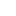喜欢 | 1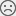不喜欢 | 0

JAVA工程师交流群 +加入

WEB/H5前端交流群 +加入•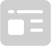资料索取
•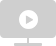答疑解惑
•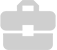技术交流
•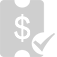职业测评
•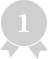面试技巧
•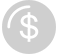高薪秘笈
JAVA30天热搜词 更多>>
X沪公网安备 31011502005948号    ICP许可  沪B2-20190160